# Examples of Solutions to Problems Involving Motion of Rigid Bodies (Part - 3) Civil Engineering (CE) Notes | EduRev

## Civil Engineering (CE) : Examples of Solutions to Problems Involving Motion of Rigid Bodies (Part - 3) Civil Engineering (CE) Notes | EduRev

The document Examples of Solutions to Problems Involving Motion of Rigid Bodies (Part - 3) Civil Engineering (CE) Notes | EduRev is a part of the Civil Engineering (CE) Course Introduction to Dynamics and Vibrations- Notes, Videos, MCQs.
All you need of Civil Engineering (CE) at this link: Civil Engineering (CE)

6.7.2 Solutions to 3D problems

Example 1: The figure shows a wheel spinning on a frictionless axle.   The axle is supported on one side (at A) by a pivot that allows free rotation in any direction.   If the wheel were not spinning, it would simply swing about A like a pendulum.   But if the angular speed is high enough, the axle remains horizontal, and the wheel turns slowly about the vertical axis.   This behavior is called ‘precession’ and is a bit mysterious – why does spin somehow hold the wheel up? The goal of this example is to explain this, and to calculate a formula for the rotation rate of the axle.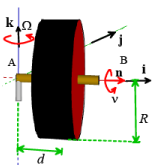We will do this by showing that steady precession satisfies all the equations of motion.

1.1 Let n be a unit vector parallel to the axle.  Consider the disk at the instant when n =i , and assume that

• the disk spins at constant rate about the axle at ν radians per second,
• the disk rotates slowly at constant rate about k at Ω radians per second

Find the angular velocity and angular acceleration at the instant shown in the figure

The angular velocity is easy – we just add the two vectors: ω =ν n +Ωk =ν i +Ωk

The angular acceleration is harder.  Both ω and Ω are constant.   But this does not mean that the angular velocity vector is constant, because the axle is rotating about the k axis.  The direction of the angular velocity is changing, even though the magnitude is not.   We can calculate the rate of change of n by using the rigid body formula

d/dt (rB −rA) = vB − vA = ω × (rB − rA)

If we choose A and B to be a unit distance apart, then (rB −rA)= n and therefore

dn/dt=ω x n

We can now calculate the angular acceleration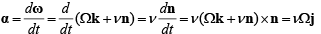1.2 Find a formula for the acceleration of the center of mass of the disk

We can use the rigid body formula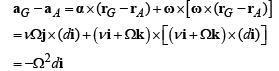A quicker way is to notice that the COM is in circular motion around A and use the circular motion formula, with the same result.

1.3 Draw a free body diagram showing the forces acting on the wheel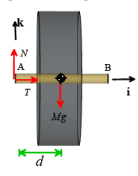1.3 Write down the equations of translational and rotational motion for the disk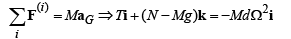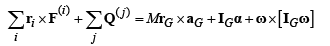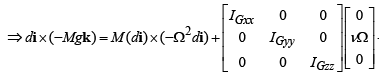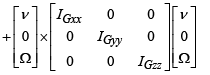Working through the cross products and the matrix-vector products we get

Mgdj = IGyyν Ωj− ( IGzz − IGxx)ν Ωj

We see that steady precession can indeed satisfy all the equations of motion.  Moreover, for a disk (or any solid of revolution) IGzz =IGyy , so we can calculate the precession rate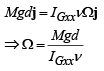Example 2: The prism shown in the figure floats in space (no gravity).  At time t=0 its faces are perpendicular to the i,j,k axes as shown.   It is then given an initial angular velocity ω = ω z0k +ωy0 j with ωy0 <<ωz 0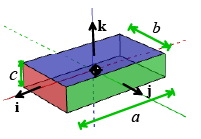(i.e. we set the body spinning about the k axis, but give it a very small disturbance) .

Investigate the nature of the subsequent motion, with both hand calculations and by writing a MATLAB script that will animate the motion of the prism.

No forces or moments act on the prism.  We can use the equations of motion

0 = MaG           0 = MrG, x aG + IGα + ω x[IGw]

The angular momentum equation can be written out explicitly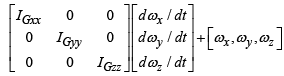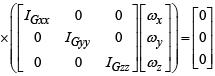(we could substitute values for IGxx ,IGyy, IGzz in terms of a,b,c and M but it is clearer to leave them)

Expanding out the matrix products and cross product gives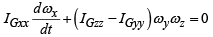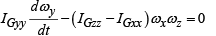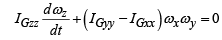At time t=0 ω is zero and ωy is small.  They might increase, but we will only consider behavior while they remain small.  In this case ωxωy is extremely small so we can assume dωz / dt ≈0 .

We can then decouple the first two equations like this:

1. Differentiate the second equation with respect to time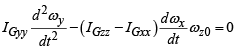2. Now we can substitute for dω x / dt using the first equation, and divide by IGyy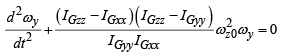This is an equation of the form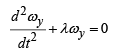We recognize this as an undamped vibration equation (case I or case II from our table of solutions).  Its solution depends on the sign of λ :

1. For λ > 0 the solution is ω y = A sin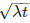+B coswhere A, B are constants.  This is stable motion - ω y remains small – this indicates that the prism will continue to spin about the k axis
2. For λ < 0 the solution is ω y = A exp+B exp(−) .   This is unstable motion - ω y will become very large. This indicates that the block will tumble.

The sign of λ is determined by the product ( IGzz −IGxx ) ( IGzz −IGyy ) .  There are three possible cases:

1. IGzz is greater than IGxx ,IGyy (the k axis has the maximum inertia).  Motion is stable
2. IGzz is less than IGxx ,IGyy (the k axis has the minimum inertia).  Motion is stable
3. IGzz is between IGxx ,IGyy .  Motion is unstable.

We can learn more about the motion by using MATLAB to solve the equations of motion for us. To do this, note that:

• Since there is no motion of the center of mass, we only need to consider rotational motion.
• We know that we can describe the orientation of the prism by the rotation tensor R and its rate of change of orientation by the angular velocity ω .  These will be our unknowns: we would like to solve for the nine components of R, and the three components of ω .
• The orientation and angular velocity are governed by the differential equations

dR/dt = WR

IGα + ω x[IGω] = 0

α = dω/dt

where IG = RIG0 RT is the rotated inertia tensor for the block, and W is the spin tensor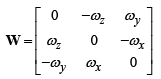We need to set up the MATLAB ‘ode’ solver to calculate R and ω as functions of time by integrating these equations.

We can store the unknown rotation matrix and the angular velocity vector in a MATLAB vector:

w =[ Rxx , Rxy , Rxz , Ryx , Ryy , Ryz , Rzx , Rzy , Rzzxyz]

We need to write a MATLAB function that will calculate the time derivatives of this vector, given its current value.  The calculation involves the following steps:

(1) Assemble the vectors ω and the rotation tensor R from the Matlab solution vector w.  Matlab has a useful function that will automatically convert a matrix to a vector, and vice-versa.  For example, R (a 3x3 matrix) can be converted to w (a 1x9 column vector) using

W(1:9) = reshape(transpose(R),[9,1]))

To transform w (as a column vector) back to R, you can use

R = transpose(reshape(w(1:9),[3,3]))

(2) Calculate the spin tensor W

(3) Calculate the rotated inertia tensor  IG = RIG0 RT (Matlab will multiply the matrices for us)

(4) Solve the equations for the angular acceleration α :

α = IG−1 (ω ×[ IGω ] .

Here IG−1 is the inverse of IG (Matlab will calculate this for us)

(5) Calculate dR / dt = WR (as a 3x3 matrix)

(6) Assemble the matlab vector dw/dt =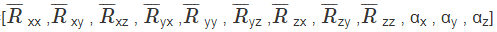This sounds complicated but actually MATLAB is great at doing this sort of calculation efficiently.  Here’s a function

function dwdt = rigid_body_eom(t,w,I0)

Rvec = w(1:9); % Rotation matrix, stored as a vector

omega = w(10:12);  % Angular velocity

R = transpose(reshape(Rvec,[3,3])); % R is now a 3x3 matrix,

II = R*I0*transpose(R); %Current inertia tensor, in fixed coord system

W = [0,-omega(3),omega(2);omega(3),0,-omega(1);-omega(2),omega(1),0];

alpha = -II\(cross(omega,II*omega)); % Angular accel

Rdot = W*R; % Rate of change of rotation matrix, as a 3x3 matrix

Rdotvec = reshape(transpose(Rdot),[9,1]);\

dwdt = [Rdotvec;alpha];

end

Now we just need to set up ode45 to integrate (numerically) the differential equation:

omega0 = [0.,0.01,1];   % Initial angular velocity

a = [4,1,2];            % Dimensions (a,b,c) of the prism

time = 20;

initial_w = [1;0;0;0;1;0;0;0;1;transpose(omega0(1:3))];

I0 = [a(2)^2+a(3)^2,0,0;0,a(1)^2+a(3)^2,0;0,0,a(1)^2+a(2)^2];

options = odeset('RelTol',0.00000001);

sol = ode45(@(t,w) rigid_body_eom(t,w,I0),[0,time],initial_w,options);

animate_rigid_body(sol,a,[0,time])

The figures below show animations of the predicted behavior for the three possible types of behavior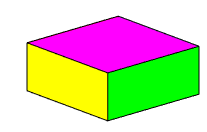I zz is the maximum inertia – rotation is stable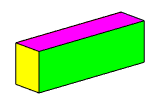I zz is the intermediate inertia – rotation is unstable (the block tumbles)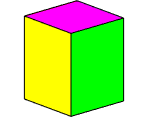I zz is the smallest inertia – rotation is stable

Offer running on EduRev: Apply code STAYHOME200 to get INR 200 off on our premium plan EduRev Infinity!

## Introduction to Dynamics and Vibrations- Notes, Videos, MCQs

20 videos|53 docs

,

,

,

,

,

,

,

,

,

,

,

,

,

,

,

,

,

,

,

,

,

;